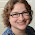## Thursday, September 18, 2014

### demo: R code to perform a voxelwise t-test

It's easy to perform a voxelwise t-test (t-test at each voxel individually). Programs for mass-univariate analysis (like SPM and fsl) of course do this (and much more), but sometimes you just want to do a simple t-test across subjects at each voxel.The demo code linked in this post does a voxelwise t-test in R. It takes as input a set of 3d NIfTI files, where each NIfTI is assumed to come from a different person, each voxel of which contains a statistic describing effect strength (for example, accuracy resulting from a searchlight analysis). The code reads the 3d NIfTI images into a 4d array (people in the fourth dimension), then performs a t-test at each voxel, saving the t-values for each voxel in a new NIfTI image. This figure shows the t-value image produced by the demo code.

### UPDATE 4 June 2021:

The original version of this post continues below. I wrote a new version of the code to 1) avoid aaply (memory usage a bit better), and 2) use the RNifti instead of oro.nifti library (RNifti functions can be dramatically faster, and have some nice features; I now use RNifti exclusively for nifti image R input/output). I also added the files to the MVPA Meanderings OSF site, under the "voxelwise" subdirectory (direct link to R script).

Both versions of this code loop through all of the image voxels, which is most assuredly not the most efficient (or foolproof) way to perform a voxelwise t-test (I would recommend 3dttest++ instead, perhaps following 3dbucket; calling afni functions directly from R can make things quite smooth for those of us that are not bash fluent). I rewrote rather than removed this demo, however, because sometimes looping through all the voxels like this is the most straightforward way to get something done.

### Original version:

Here is the key part of the code. The 4d array big contains the 3d statistic images for each person (subjects are the 4th dimension of the array). Then the plyr function aaply calls my little getT function (below and in the code file), which calculates the t-value at each voxel (ie over the subjects), creating a 3d array of t-values.

`````` # function to calculate the t-test at each voxel and return the t value
getT <- function(x) {
# can't do a t-test if variance is zero, so check before trying.
if (var(x) == 0) {
stat <- NA;
} else {
stat <- t.test(x, alternative="greater", mu=0.5)\$statistic;
}
return(stat)
}
# plyr function aaply calls getT at each voxel in the 4d array big,
# creating a 3d output array of t-values (what getT returns)
t.img <- aaply(big, c(1,2,3), getT);
``````

1.hi thank you a lot for this post!I need to do a paired t test though and I find really hard implementing the function: any suggestion? thanks a lot again

1.If you put the pairs of x into the vector y, then it's t.test(x, y, alternative="greater", mu=0.5, paired=TRUE);

2.I'm sorry but how can I extract recursively the corresponding paired value of x from the other dataset (let's say we have only two datasets stacked together)? I feel my question is really trivial but I'm stuck for days finding a solution..

3.Not sure of a one-line solution with aaply, but it should work with massive loops. For example, if you have paired people in two 4D images (so the 4th dimension is the same size in each image), you could run the test on each voxel sequentially, like this, for the voxel at i,j,k:
x <- img1[vox.i, vox.j, vox.k,];
y <- img2[vox.i, vox.j, vox.k,];
t.test(x, y, alternative="greater", mu=0.5, paired=TRUE);

4.Hi Jo thanks for replying. I did this:
for (i in seq_len(dim(big))) {
for (j in seq_len(dim(big))) {
for (k in seq_len(dim(big))) {
x <- big[i, j, k,];
y <- big[i, j, k,];
stat[i,j,k] <- t.test(x, y, paired=TRUE,var.equal=T)\$statistic;
}
}
}

but it is giving me all NaNs in the output for some reason...

5.I don't know the seq_len function, but probably your i, j, k aren't being set to in-brain integers. Regardless, it's always easier to debug out of a loop ... I'd check the values that are going into x and y for a single voxel, that the t-test is reasonable, etc., before scaling up to whole-brain.

6.Thank you. I checked and the values are correct.One problem I think is that I have only two images (same subject before and after a condition): I guess t.test() does not have enough values.What is your opinion? thanks

2.Hi! Thanks a lot for this post. I wanted to use it to run a t-test on accuracy maps from a searchlight analysis. Unfortunately the files have been moved. Is the code still accessible somewhere? Or do you have other suggestions how run the group level t-test on accuracy maps?

1.the dropbox sharing settings changed, which broke the links ... I just updated it, so give it a try again.